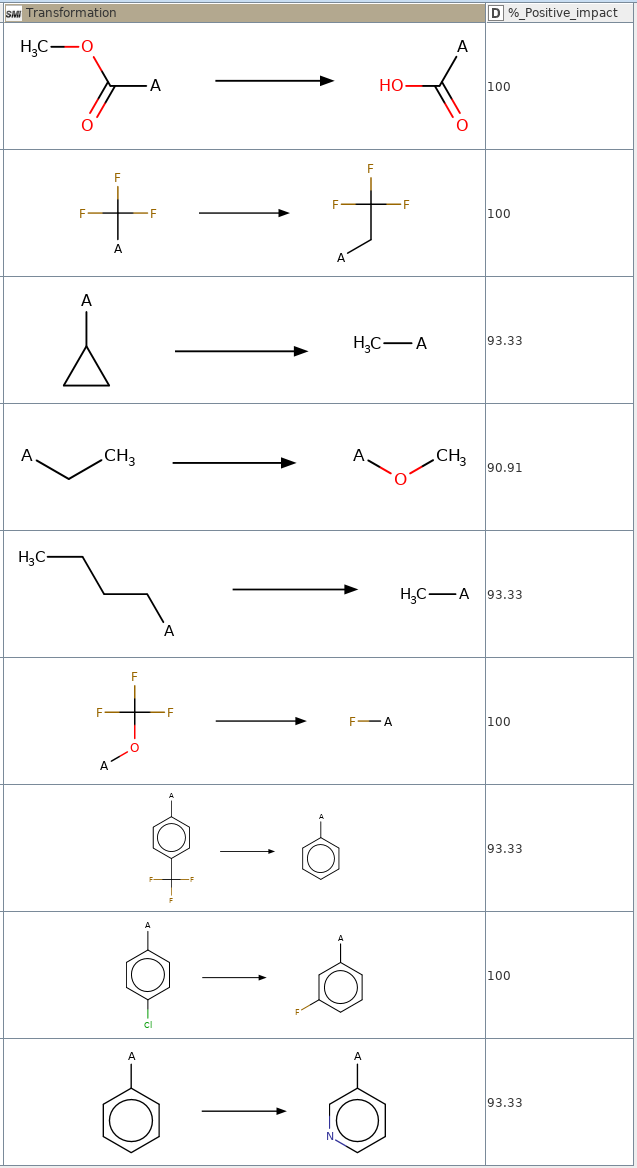# Impact of chemical transformations on the hERG inhibition¶

The inhibition of the human ether-a-go-go (hERG) ion channel may cause QT interval prolongation, which eventually can result in torsades de pointes (TdP) and even death. Hence cardiotoxicity caused by the inhibition of hERG is a major liability within the drug development process. To avoid such a severe adverse effect, it makes good sense to understand which chemical transformation can have a positive impact on the hERG inhibition.

hERG_Matched_Molecular_Pair_analysis is a Knime workflow capable of fetching hERG data from the ChEMBL database. A Matched Molecular Pair analysis is then performed to allow a bar chart data visualisation. The positive, negative or neutral impact percentage of each transformation can be visualize as a stacked bar chart.

 Journal of Cheminformatics volume 11, Article number: 9 (2019)

In :
```import pandas as pd
import knime

%matplotlib inline
import numpy as np
import matplotlib.pyplot as plt
import matplotlib

matplotlib.style.use('ggplot')
```

In [ ]:
```knime.executable_path = "/opt/knime_4.1.1/knime"
```

In :
```workspace = "/mnt/DATA/knime_workspace_production"
```

Now pick the Knime workflow

In :
```workflow = "hERG_MMP_analysis_with_ChEMBL_data_Czodrowski_AG_5_min_of_fame"
knime.Workflow(workflow_path=workflow,workspace_path=workspace)
```
Out:

Run the Knime workflow

In :
```with knime.Workflow(workflow_path=workflow,workspace_path=workspace) as wf:
wf.execute()
```

Get the data from Knime as a Pandas DF

In :
```df = wf.data_table_outputs
```

Start from here if Knime is not installed on your computer.

In :
```#df = pd.read_csv ('./data/hERG_MMP_ChEMBL.csv')
```

Reorganise the DF for plotting

In :
```df = df.sort_values(by=['Positive_impact']).set_index('Transformation')
```

Plot the impact of each chemical transformation the hERG inhibition.

Applying a transformation with a high positive impact in % might have a high likelyhood of decreasing the hERG liability of a molecule for which such a transformation was applied thus reducing the cardiotoxicity of this molecule.

In :
```ax = df.plot.barh(figsize=(10, 250), stacked=True, title="Chemical transformation impact in %", fontsize=16)
```Getting back "Transformation" as a Pandas column

In :
```df = df.reset_index(level='Transformation')
```
In :
```df.head(10)
```
Out:
Transformation Negative_impact Neutral_impact Positive_impact
0 *c1ccccn1>>*c1ccc(F)cc1 90.00 10.0 0.00
1 *CCC>>*CCCC 100.00 0.0 0.00
2 *C(=O)O>>*C(=O)OC 100.00 0.0 0.00
3 *F>>*OC(F)(F)F 100.00 0.0 0.00
4 *c1cccc(F)c1>>*c1ccc(Cl)cc1 100.00 0.0 0.00
5 *c1cccnc1>>*c1ccccc1 93.75 0.0 6.25
6 *c1ccccc1>>*c1ccc(C(F)(F)F)cc1 93.75 0.0 6.25
7 *C>>*CCCC 92.86 0.0 7.14
8 *CC(F)(F)F>>*C(F)(F)F 90.91 0.0 9.09
9 *c1ccc(F)cc1>>*c1ccc(C)cc1 90.91 0.0 9.09

The table le below was obtained from the Knime workflow:In [ ]:
```
```